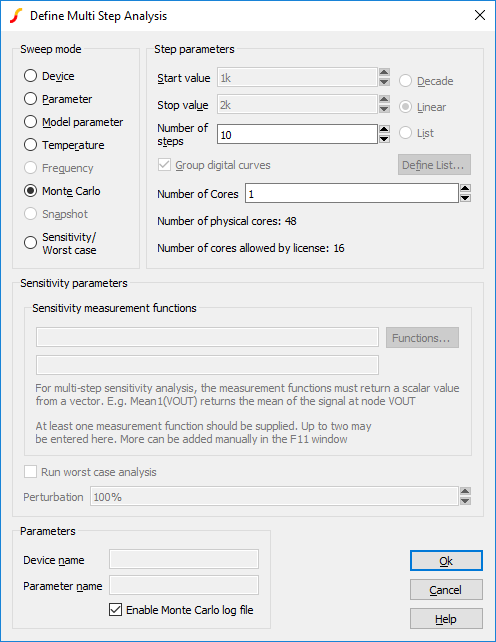# Running Sensitivity and Worst-case

In this topic:

## Overview

As with Monte Carlo, there are both Multi-step and single-step variants of sensitivity and worst-case analyses. Single-step can be applied to AC, DC, Noise and transfer function analyses; multi-step can be applied to the same analyses in addition to transient analysis.

The example shown above (Sensitivity/Worst-case Example) is an example of a multi-step analysis and is the most commonly used.

Single-step analysis can be used when the measurement being performed can be done on a single point. For example, the gain of a linear amplifier at a single frequency can be obtained from a single point of an AC analysis. Similarly the, DC offset of an amplifier can be evaluated from a single point of a DC analysis. If these are the quantities being studied then a single-step sensitivity analysis may be used with major speed advantages.

## Setting up a Multi-step Sensitivity Analysis

1. Select schematic menu Simulator > Choose Analysis.... Select the AC, DC, Noise, Transient or TF tab as required.
2. Define the analysis as required.
3. In the Monte Carlo and Multi-step Analysis group, check the Enable multi-step box then press the Define... button. This will open:-4. In the Sweep mode group, select Sensitivity/Worst-case.
5. In the Sensitivity parameters group, enter at least one sensitivity measurement function. For multi-step analyses, this must be a function that returns a scalar value from a vector. For example, the function mean1() returns the mean of its argument.
6. You can set the Number of Cores to a number large than 1 if your system and license permit it. This will usually substantially speed up the run except for small circuits. For more information see Using Multiple Cores for Multi-step Analyses.

## Setting up a Single Step Sensitivity Analysis

1. Select schematic menu Simulator > Choose Analysis.... Select the AC, DC, Noise or TF tab as required.
2. In the Sweep Parameters group, press the Define... button.
3. In the Sweep Mode group select Sensitivity/Worst-case.
4. In the Sensitivity parameters enter at least one sensitivity measurement function. For single step, the analysis needs to obtain a measurement for each simulation point, so you must enter an expression that returns a vector from the sweep. This is usually simply the value of a node voltage or current. For AC analyses, you must apply a complex-real conversion function, e.g, db(), mag(), phase(), real() or imag().
5. For AC, Noise and TF, you must also supply a value for Frequency.
6. If you wish a worst-case analysis to be performed after the sensitivity analysis, check the Run worst-case analysis box.